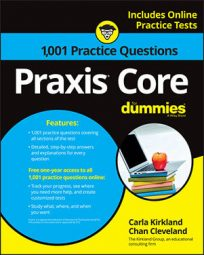##### Praxis Core: 1,001 Practice Questions For DummiesPrisms weren't invented just to divide light into beautiful rainbows; they're also useful for inducing painful headaches when you're asked to find their surface area or volume on the Praxis Core exam.

Swallow a headache tablet and try the following practice questions. In the first one, you have to find the surface area of a right rectangular prism. In the second one, you're asked to find the volume of a triangular prism.

## Practice questions

1. The following figure is a right rectangular prism. What is its surface area?

A. 266 m2 B. 144 m2 C. 246 m2 D. 432 m2 E. 348 m2

2. A triangular prism has two triangular bases that both have an area of 19 square units. The prism has a height of 8 units. What is the volume of the prism?

A. 604 cubic units B. 76 cubic units C. 27 cubic units D. 152 cubic units E. 228 cubic units

1. The correct answer is Choice (E).

The surface of any right prism is Ph + 2B, which is the product of the perimeter of a base and its corresponding height, plus twice the area of the base. Any face of a right rectangular prism can be considered a base, and what you use for height must be the measure of a segment perpendicular to the face you decided to consider a base. For example, if you consider an 8 m-by-9 m face a base for the right rectangular prism in this problem, you must consider 6 m to be the height. In that case, the other 8 m-by-9 m face is also a base.

The surface area of the right rectangular prism is 348 m2.

2. The correct answer is Choice (D).

The volume of any prism is Bh, or base area times the height:

The volume of the prism is 152 cubic units.